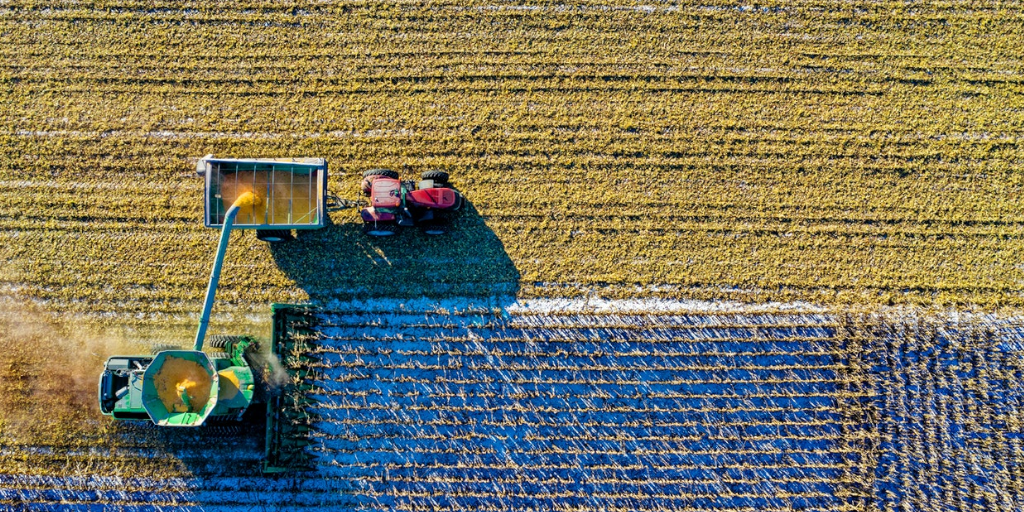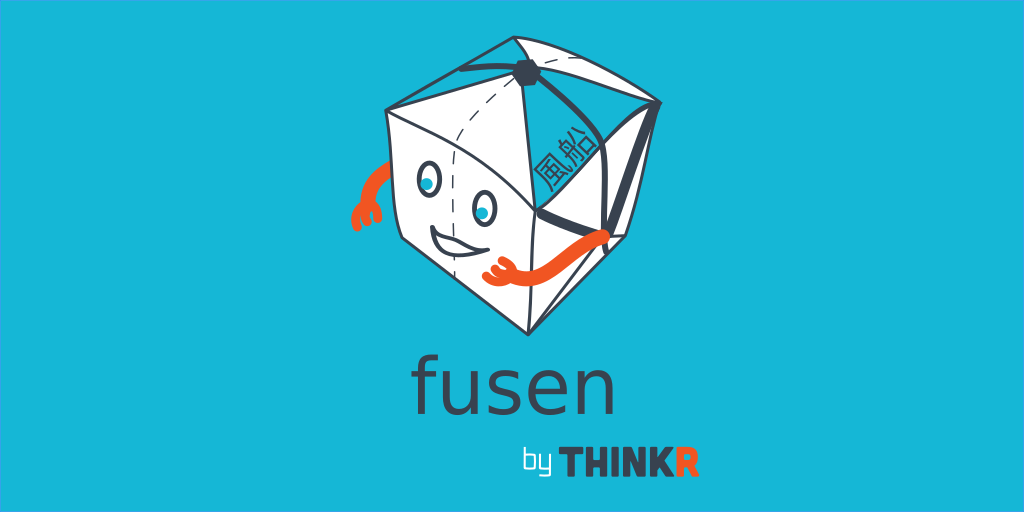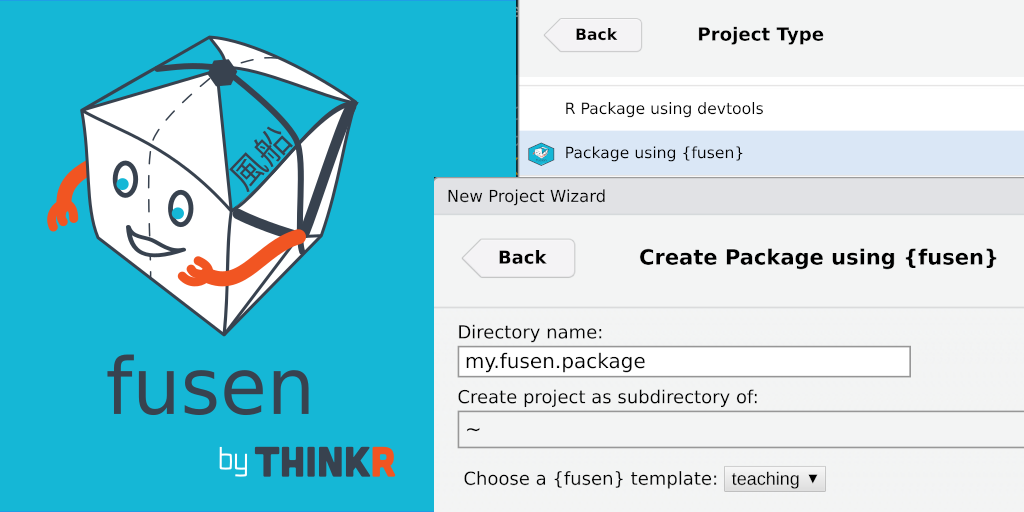# Row-wise operations with the {tidyverse}Tags : data, tidyverse, tips
Date :

We are often asked how to perform row-wise operations in a `data.frame` (or a `tibble`) the answer is, as usual, “it depends” 🙂

Let’s look at some cases that should fit your needs.

``library(tidyverse)``

Let’s make an example dataset:

``````base <- tibble::tibble(
a = 1:10,
b = 1:10,
c = 21:30
base``````
``````## # A tibble: 6 × 3
##       a     b     c
##   <int> <int> <int>
## 1     1     1    21
## 2     2     2    22
## 3     3     3    23
## 4     4     4    24
## 5     5     5    25
## 6     6     6    26``````

Let’s say we want to add a `new` column whose value will depend on the content, per row, of columns `a`, `b` and `c` of our `base` example

Like this:

``````# A tibble: 6 x 4
a     b     c new
<int> <int> <int> <chr>
1     1     1    21 a equals 1
2     2     2    22 other case
3     3     3    23 other case
4     4     4    24 other case
5     5     5    25 c equals 25
6     6     6    26 other case``````

## With `case_when()`

``````base %>%
mutate(
new = case_when(
a == 1 ~ "a equals 1",
c == 25 ~ "c equals 25",
TRUE ~ "other case"
)
)``````
``````## # A tibble: 6 × 4
##       a     b     c new
##   <int> <int> <int> <chr>
## 1     1     1    21 a equals 1
## 2     2     2    22 other case
## 3     3     3    23 other case
## 4     4     4    24 other case
## 5     5     5    25 c equals 25
## 6     6     6    26 other case``````

`case_when()` is nice, it’s much more readable than nested `ifelse()`, but it can quickly become more complex.
So let’s create a function which, depending on the values of `a`, `b`, `c`, returns the expected value.

Depending on the case (and your skills) you will sometimes have a vectorized function and sometimes a non-vectorized function. It is always better to create a vectorized function, but it is not always possible.
A vectorized function is a function that can be directly applied to a set of vectors and that returns a response vector.

An example of a vectorized function that repeats the operations of the previous `case_when()`:

``````vectorised_function <- function(a, b, c, ...){
ifelse(a == 1 , "a equals 1",
ifelse(c == 25 , "c equals 25",
"other case"
))
}
vectorised_function(a = 1, c = 25, b = "R")``````
``##  "a equals 1"``
``vectorised_function(a = c(1, 1, 3), c = 27:25, b = "R")``
``##  "a equals 1"  "a equals 1"  "c equals 25"``

Here is the “same” function, but not vectorized:

``````non_vectorised_function <- function(a, b, c, ...){
if ( a == 1  ) { return("a equals 1") }
if ( c == 25 ) { return("c equals 25") }
return("autre")
}
non_vectorised_function(a = 1, c = 25, b = "R")``````
``##  "a equals 1"``
``non_vectorised_function(a = c(1, 1, 3), c = 27:25, b = "R") # ne fonctionne pas``
``````## Warning in if (a == 1) {: la condition a une longueur > 1 et seul le
## premier élément est utilisé``````
``##  "a equals 1"``

## With a vectorized function

This is the simplest case, and the fastest too.
You can use it as is in a `mutate()` :

``````base %>%
mutate(
new = vectorised_function(a = a, b = b, c = c)
)``````
``````## # A tibble: 6 × 4
##       a     b     c new
##   <int> <int> <int> <chr>
## 1     1     1    21 a equals 1
## 2     2     2    22 other case
## 3     3     3    23 other case
## 4     4     4    24 other case
## 5     5     5    25 c equals 25
## 6     6     6    26 other case``````

## With a NON vectorized function

The result returned by a `mutate()` is not correct (the first value returned is repeated…)

``````base %>%
mutate(
new = non_vectorised_function(a = a, b = b, c = c)
)``````
``````## Warning in if (a == 1) {: la condition a une longueur > 1 et seul le
## premier élément est utilisé``````
``````## # A tibble: 6 × 4
##       a     b     c new
##   <int> <int> <int> <chr>
## 1     1     1    21 a equals 1
## 2     2     2    22 a equals 1
## 3     3     3    23 a equals 1
## 4     4     4    24 a equals 1
## 5     5     5    25 a equals 1
## 6     6     6    26 a equals 1``````

So let’s change our strategy.

### With `rowwise()`

`rowwise()` is back in the {dplyr} world and is specifically designed for this case:

``````base %>%
rowwise() %>%
mutate(
new = non_vectorised_function(a = a, b = b, c = c)
)``````
``````## # A tibble: 6 × 4
## # Rowwise:
##       a     b     c new
##   <int> <int> <int> <chr>
## 1     1     1    21 a equals 1
## 2     2     2    22 autre
## 3     3     3    23 autre
## 4     4     4    24 autre
## 5     5     5    25 c equals 25
## 6     6     6    26 autre``````

### With `pmap()`

``````base %>%
mutate(
new = pmap_chr(list(a = a, b = b, c = c), non_vectorised_function)
)``````
``````## # A tibble: 6 × 4
##       a     b     c new
##   <int> <int> <int> <chr>
## 1     1     1    21 a equals 1
## 2     2     2    22 autre
## 3     3     3    23 autre
## 4     4     4    24 autre
## 5     5     5    25 c equals 25
## 6     6     6    26 autre``````

## Bonus with `Vectorize()`

The `Vectorize()` function allows to vectorize a function…
It’s a bit of a cheat, but it can help 🙂

``````base %>%
mutate(
new = Vectorize(non_vectorised_function)(a = a, b = b, c = c)
)``````
``````## # A tibble: 6 × 4
##       a     b     c new
##   <int> <int> <int> <chr>
## 1     1     1    21 a equals 1
## 2     2     2    22 autre
## 3     3     3    23 autre
## 4     4     4    24 autre
## 5     5     5    25 c equals 25
## 6     6     6    26 autre``````

## Row-wise operations are yours!

Experiment and tell us what your practices are!

To go further: https://dplyr.tidyverse.org/articles/rowwise.htmlCodeur fou, formateur et expert logiciel R#### {fusen} v0.4 – 14 developers tips and tricks

2022-05-03 / Sébastien Rochette#### ‘fusen’ v0.3: Better project templates, grouped functions and numerous other enhancements

2021-12-19 / Sébastien Rochette

07/06/2022

13/09/2022

13/09/2022

19/03/2022

02/02/2022

18/11/2021

par ThinkR